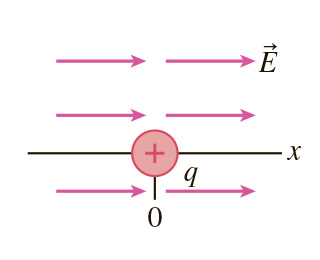# Problem: As shown in (Figure 1) , a q = 9.0 nC charge sits at x = 0 in a uniform E = 4500 N/C electric field directed to the right.Part A. At what point along the x-axis would a proton experience no net force? Express your answer to two significant figures and include the appropriate units.Part B. At what point along the x-axis would an electron experience no net force? Express your answer to two significant figures and include the appropriate units.

###### FREE Expert Solution

The electrostatic force between two charges q1 and qis:

$\overline{){\mathbf{F}}{\mathbf{=}}\frac{\mathbf{k}{\mathbf{q}}_{\mathbf{1}}{\mathbf{q}}_{\mathbf{2}}}{{\mathbf{r}}^{\mathbf{2}}}}$, where is Coulomb's constant and is the distance between q1 and q2.

If the charges have opposite signs, the force between them will be attractive and repulsive if the charges have the same sign.

The force on the positive charge due to the electric field is directed along the direction of the electric field.

Force on the negative charge due to electric field is directed opposite to the electric field direction.

96% (7 ratings)###### Problem Details

As shown in (Figure 1) , a q = 9.0 nC charge sits at x = 0 in a uniform E = 4500 N/C electric field directed to the right.Part A. At what point along the x-axis would a proton experience no net force? Express your answer to two significant figures and include the appropriate units.

Part B. At what point along the x-axis would an electron experience no net force? Express your answer to two significant figures and include the appropriate units.